×
Get Full Access to OleMiss - ECON 202 - Class Notes - Week 2
Get Full Access to OleMiss - ECON 202 - Class Notes - Week 2

×

OLEMISS / OTHER / ECON 202 / what is The Scientific Method of Economics?

# what is The Scientific Method of Economics? Description

ECON202 CHAPTER 2 Notes Cheng

## what is The Scientific Method of Economics?• Economics is a social science.

• The Scientific Method of Economics –

o Observe economic phenomenon

o Formulate a theory/model that is testable

o Collect data and test the theory through empirical  analysis

o Revise the theory if it fails to explain the data well ▪ Example: Do couples have more children when  they earn more? This is a testable question that

phenomenon.

• How does economics differ from other sciences? o While most sciences are based on facts, economics  has a basis in assuming human behavior is rational. ▪ Example: How does economics explain why the  \$100 bill on the street will disappear? Assuming

there is no wind, a person that happens upon a

\$100 bill will perform a cost/benefit analysis. Cost  of picking up the \$100? None, unless you feel

## How does economics differ from other sciences?guilty about it. Benefit of picking up \$100?

Well….you get an extra \$100 with little to no work  exerted.

• Economic models/theories – when trying to understand  economics, the answer to most economic phenomena is  knowing the causation of such phenomena. Economists  are required to simplify complex human behaviors by… o Assuming away unnecessary details

o Understanding the simplest scenario first before  adding complexities to it

o Making assumptions to make things simple, then  relaxing (or specifying) these simple assumptions when analyzing complicated scenarios If you want to learn more check out uic econ 120 final exam

Reality (Human  Behavior)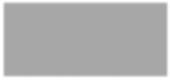## what are the 3 ways to describe an economic model/theory?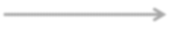lots of assumptions less assumptions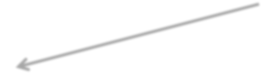few assumptions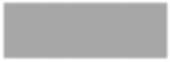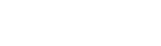Simplest Model We also discuss several other topics like vaswlar

Moderate Model Complicated  Model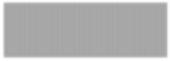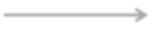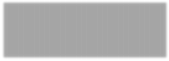If you want to learn more check out uga pe classes

o The balance of economic models is extremely  important.

▪ If a model is overbroad (meaning it includes too  many moving variables and not enough

assumptions), an economist task of explaining a  phenomenon will be almost impossible.

▪ If a model is oversimplified (meaning it includes  too many assumptions), an economist will not

come to an accurate conclusion when testing his  model/theory.

▪ Example: Say you need a map from your house to  your next class. Do you need a map that includes  all of the trees, lakes, buildings, etc all along your  route? (Probably not, this would be overbroad.)  What if I gave you a map with a squiggly line from  point A to point B? (Not helpful either, because  it’s oversimplified.)

o There are 3 ways to describe an economic  model/theory. Most often, you’ll want to use all three  methods in creating a model.

▪ Verbal description (in plain words) Don't forget about the age old question of world civilization study guide

▪ Diagrams/tables/graphs

▪ Mathematical equations

▪ Example: The Law of Demand

• Verbal description – Consumers buy less  when the price of a good goes up (and vice  versa)

• Graphical description –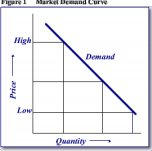• Mathematical equation (example) –

Quantity Demanded = 100 – (5 * Price)

• Production Possibilities Frontier (PPF)

o This economic model explains efficient production  possibilities, or the allocation of 100% of available  resources in the most profitable/beneficial way • It will demonstrate how a specific choice of

action will result in an efficient, inefficient, or

unfeasible manner

o Efficient – economy uses ALL available  We also discuss several other topics like meteorology schools in texas

resources to produce a combination of

2 goods; the PPF is the set of all

efficient output combinations

o Inefficient – economy does NOT have

to use all of its resources to produce it,

meaning there are resources leftover;

this point would be plotted BELOW the

PPF line on a graph)

o Infeasible (not possible) – economy

cannot produce the combination of

goods, even if all resources are used

(this point would be plotted ABOVE the

PPF line on a graph)

o Example: Consider an economy that uses the scarce  resource (labor, measured by labor hours) to produce  computers and wheat. Assume the economy has  50,000 labor hours per month available for production. In addition, producing one computer requires 100  hours labor; producing one ton of wheat requires 10  hours labor. Below are five production plans in terms  of allocating the 50,000 labor hours:

• A. 50,000 hours in producing computers + 0  hour in producing wheat

• B. 40,000 hours in producing computers +  10,000 hours in producing wheat

• C. 25,000 hours in producing computers +  25,000 hours in producing wheat

• D. 10,000 hours in producing computers +  40,000 hours in producing wheat

• E. 0 hour in producing computers + 50,000  hours in producing wheat

▪ From the information above, we know that we  can produce 500C/0W, 400C/1000W,

250C/2500W, 100C/4000W, or 0C/5000W. This is  the PPF line on the graph, because all labor hours  would be used in each output scenario.

• Say you wanted to produce 100C and

3500W. This would be inefficient, because

there would be leftover labor hours that

were not used. The point would fall below  PPF.

• Say you wanted to produce 300C and  3500W. This would be infeasible, because  you don’t have enough labor hours available.  The point would fall above PPF. Remember,  resources are scarce!

Chapter 2 Homework  Questions:

1. One thing economists do to help them understand how the real  world works is… make assumptions.

2. Economists make assumptions to… make a complex world  easier to understand.

3. An economic theory about international trade that is based on  the assumption that there are only two countries trading two  goods… can be useful in helping economists understand the  complex world on international trade involving many countries  and many goods.

4. Which of the following statements about economic models is  correct?... Because economic models omit many details, they  allow us to see what is truly important.

5. Suppose a nation is currently producing at a point inside its  production possibilities frontier. We know that... the nation is  not using all of its available resources.

6. If this economy devotes all of its resources to the production  of clocks, then it will produce… 16 clocks and 0 candles.

7. This economy has the ability to produce at which points?... A,  B, C, F, G

8. If the resource is not fully utilized (e.g., unemployment), could  this economy produce at which point(s)?... C, F, G

9. What is the opportunity cost of moving from

point A to point B?... 15 candles.

10. For this economy, as more and more clocks are produced, the  opportunity cost of an additional clock produced (in terms of  candles)… increases.

11. The movement from point B to point D could NOT be caused  by… unemployment.

12. Which of the following events would explain the shift of the  production possibilities frontier from A to B?... The economy  experienced a technological advance in the production of  books.

13. When a production possibilities frontier is bowed outward,  the opportunity cost of producing an additional unit of a good… (not sure… will update when I know the answer!)

14. If the production possibilities frontier is bowed

outward, then "?" could be… (not sure, will update when I know  the answer!)

15. Production possibilities frontiers are usually bowed outward.  This is because… it reflects the fact that the opportunity cost  of producing a good decreases as more and more of that good  is produced.

16. Which of the following is an example of a positive, as  opposed to normative, statement?... When the quantity of  money grows rapidly, inflation is a predictable consequence.

Page ExpiredIt looks like your free minutes have expired! Lucky for you we have all the content you need, just sign up here